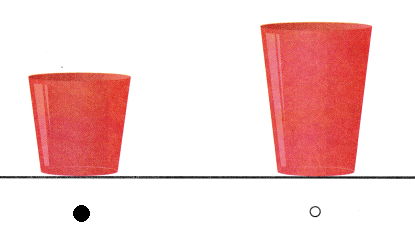# Texas Go Math Kindergarten Lesson 19.3 Answer Key Compare Heights

Refer to our Texas Go Math Kindergarten Answer Key Pdf to score good marks in the exams. Test yourself by practicing the problems from Texas Go Math Kindergarten Lesson 19.3 Answer Key Compare Heights.

## Texas Go Math Kindergarten Lesson 19.3 Answer Key Compare Heights

Essential Question
How can you compare the heights of two objects?

Explore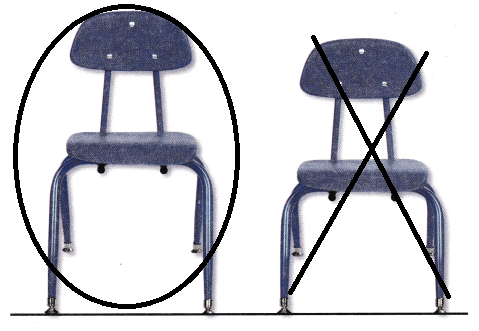Explanation:
The taller chair is circled and the shorter chair is crossed
The first chair is taller than the second chair.

Directions
Look at the choirs. Compare the heights of the two chairs Use the words taller than, shorter than, or about the same height to describe the heights. Draw around the taller chair. Draw on X on the shorter chair.

Share and Show

Question 1.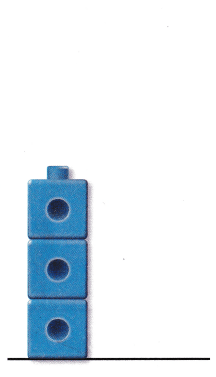Explanation:
A yellow cube tower is drawn that is longer than the given blue tower

Question 2.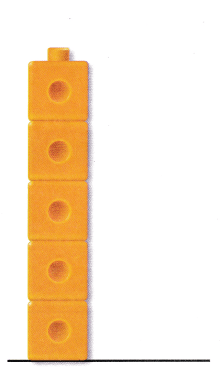Explanation:
A yellow cube tower is drawn that is longer than the given orange tower

Directions 1-2. Make a cube tower that is taller than the cube tower shown. Draw and color the cube tower.

Question 3.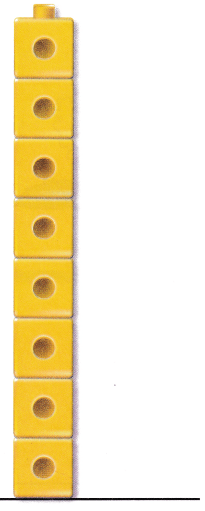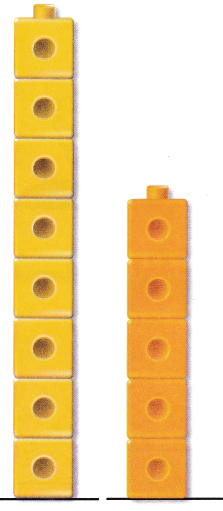Explanation:
A orange cube tower is drawn that is shorter than the given yellow tower

Question 4.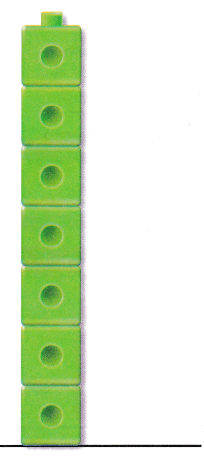Explanation:
A blue cube tower is drawn that is shorter than the given green tower

Directions
3-4. Make a cube tower that is shorter than the cube tower shown. Draw and color the cube tower.

Problem Solving

Question 5.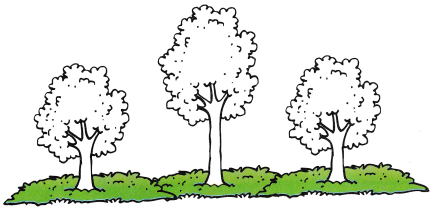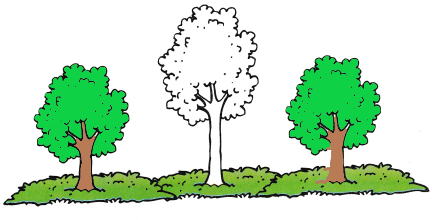Explanation:
The same height tree are colored with green
the first and last trees are of same height.

Question 6.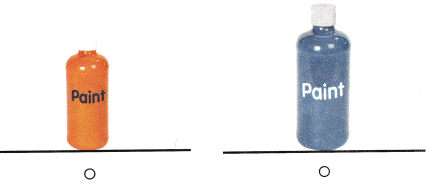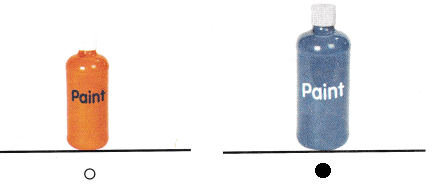Explanation:
The blue paint bottle is taller than the orange paint bottle
so, marked the blue paint bottle.

Directions
5. Color the trees that are about the some height. 6. Choose the correct answer. Which container is taller?

Home Activity

• Have your child find two objects such as plastic toys or stuffed animals. Have him or her place the objects side by side to compare the heights. Ask your child which object is taller and which object is shorter.

### Texas Go Math Kindergarten Lesson 19.3 Homework and Practice

Question 1.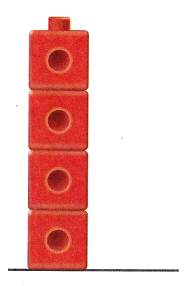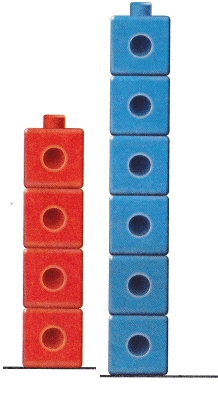Explanation:
A blue cube tower is drawn that is longer than the given red tower

Question 2.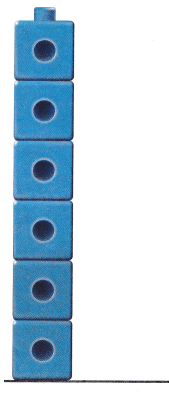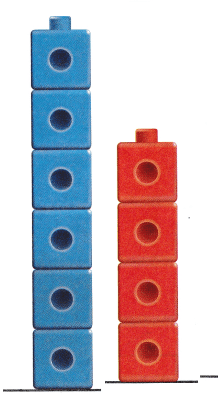Explanation:
A red cube tower is drawn that is shorter than the given blue tower

Directions
1. Draw and color a cube rower that is taller than the cube tower shown.
2. Draw and color a cube tower that is shorter than the cube tower shown.

Texas Test Prep

Lesson Check

Question 1.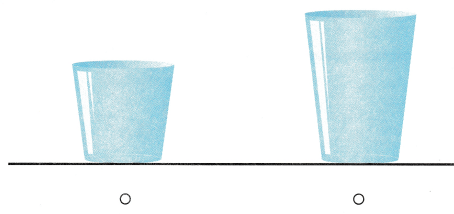Explanation:
The second blue glass is taller than the first red glass
so, marked the second blue glass.

Question 2.